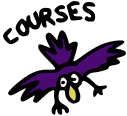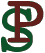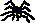Math 4680/5680, Topics in Logic and ComputationWinter 2017 Peter Selinger "Introduction to Mathematical Logic"

### Course Information

 See the Syllabus.

### Announcements

 Final exam (posted Mar 22). The final exam will be on Tuesday, April 18, at 9:30, in our usual classroom, Chase 319. Midterm (posted Feb 23). The midterm will be in class on March 8. Midterm (posted Feb 17). We can't have the midterm on March 6. I propose March 8. Please let me know right away if you have a conflict that day. Office hours (posted Feb 7). My current office hours are Mondays 10:30-11:30, or by appointment. Practice problems (posted Jan 16). Please practice natural deduction derivations, using problems 9, 12, 15, 18, ..., 39 from Handout 1. Welcome to the course (posted Jan 9). Here, you will find up-to-date information, including announcements, homework assignments, any handouts, etc.

### Suggested Topics for Student Presentations

Here are some possible topics for graduate student presentations.
• Decidability [taken]. What does it mean for a function to be computable? What does it mean for a question to be decidable? Recursive and recursively enumerable sets.
• Analytic tableaux. Analytic tableaux are another proof system for first-order logic, well suited for automatic proof search. Reference: Raymond Smullyan, "First Order-Logic". Dover Publications, 1968, 1995.
• Non-standard models of analyis [taken]. Explore non-standard models of the real numbers. In such models, there exist infinitesimals, and one can do calculus without using limits.
• Formal mathematics. Report on efforts to formalize real-world mathematics in computer-checkable form. Reference: Thomas C. Hales, "Formal Proof", Notices of the AMS, 55(11):1370--1380, December 2008.
• Type theory. Type theory is an alternative to set theory as a practical foundation of mathematics.
• Intuitionistic logic [taken]. Intuitionistic logic is concerned with constructive proofs: any proof of existence should yield an actual witness. Explore the laws of propositional intuitionistic logic and Kripke semantics. Reference: A. S. Troelstra and D. van Dalen, "Constructivism in mathematics: an introduction", North-Holland, 1988.
• Sequent calculus. Sequent calculus is an alternative proof system for first-order logic. It has a particularly attractive symmetry. Reference: Jean Gallier, "Constructive Logics. Part I: A Tutorial on Proof Systems and Typed lambda-Calculi". Theoretical Computer Science, 110(2), 249-239 (1993).
• Zero-one law [taken]. The probability that a first-order sentence holds in a random structure of size n either goes to 0 or to 1, as n goes to infinity. Reference: Fagin 1976.

### Homework Assignments

 Homework 1, due Jan 27. 2.2 #1, 2; 2.4 #2; 2.6 #5; Handout 1, #36–38, 42–44. Homework 2, due Feb 6. 2.1 #2; 2.5 #1, 3, 4, 9. Homework 3, due Feb 17. 3.4 #1, 4, 5, 7. Homework 4, due Mar 3. Handout 2, #1(h), (j); #2(w); #4(e), (f), (h). Homework 5, due Apr 10. Handout 4, #1, 3–6.

### Handouts

 Handout 1 (Jan 16) [pdf]: Problems for propositional logic Handout 2 (Feb 15) [pdf]: Natural deduction for quantifiers Handout 3 (Mar 15) [pdf]: Lecture Notes: Consequences of completeness for first-order logic Handout 4 (Apr 3) [pdf]: Problems

To Peter Selinger's Homepage:Peter Selinger / Department of Mathematics and Statistics / Dalhousie University
selinger@mathstat.dal.ca / PGP key